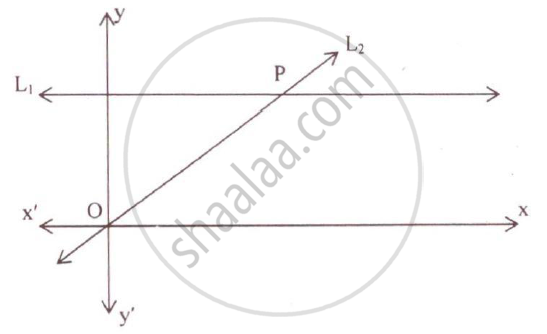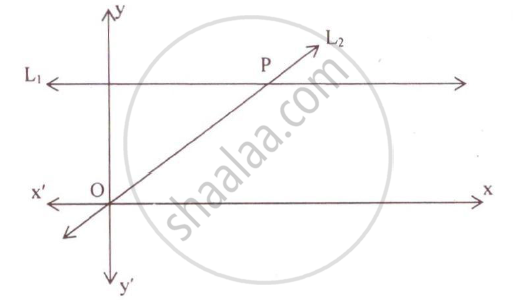Share

# Given the Equation of Line L, is Y = 4. (1) Write the Slope of Line L2, If L2, is the Bisector of Angle O. (2) Write the Co–Ordinates of Point P. (3) Find the Equation of L2. - ICSE Class 10 - Mathematics

#### Question

Given the equation of line L, is y = 4.
(1) Write the slope of line L2, if L2, is the bisector of angle O.
(2) Write the co–ordinates of point P.
(3) Find the equation of L2.#### Solution

The equation of the line L1 is y = 4.
It is given that L2 is the bisector of angle O and ∠O = 90˚.
Thus, the line L2 makes an angle of 45˚ with the x-axis.
Thus, slope of line L2 = tan 45˚ = 1The line L2 passes through (0, 0) and its slope is 1. So, its equation is given by
y – y1 = m(x – x1)
y – 0 = 1(x – 0)
y = x
Now, the point P is the point of intersection of the lines L1 and L2.
Solving the equations y = 4 and x = y, we get x = y = 4
Thus, the coordinates of the point P are (4, 4).

Is there an error in this question or solution?

#### APPEARS IN

2010-2011 (March) (with solutions)
Question 11.3 | 4.00 marks
Solution Given the Equation of Line L, is Y = 4. (1) Write the Slope of Line L2, If L2, is the Bisector of Angle O. (2) Write the Co–Ordinates of Point P. (3) Find the Equation of L2. Concept: Equation of a Line.
S